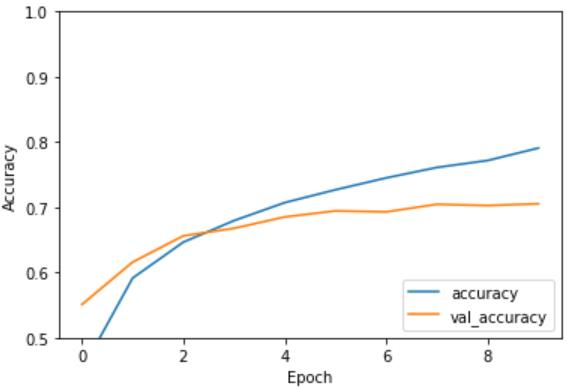# How can Tensorflow be used to evaluate a CNN model using Python?

A convolutional neural network can be evaluated using the ‘evaluate’ method. This method takes the test data as its parameters. Before this, the data is plotted on the console using ‘matplotlib’ library and ‘imshow’ methods.

Convolutional neural networks have been used to produce great results for a specific kind of problems, such as image recognition.

We are using the Google Colaboratory to run the below code. Google Colab or Colaboratory helps run Python code over the browser and requires zero configuration and free access to GPUs (Graphical Processing Units). Colaboratory has been built on top of Jupyter Notebook.

print("Plotting accuracy versus epoch")
plt.plot(history.history['accuracy'], label='accuracy')
plt.plot(history.history['val_accuracy'], label = 'val_accuracy')
plt.xlabel('Epoch')
plt.ylabel('Accuracy')
plt.ylim([0.5, 1])
plt.legend(loc='lower right')
print("The model is being evaluated")
test_loss, test_acc = model.evaluate(test_images,test_labels, verbose=2)
print("The accuracy of the model is:")
print(test_acc)

Code credit: https://www.tensorflow.org/tutorials/images/cnn

## Output

Plotting accuracy versus epoch
The model is being evaluated
313/313 - 3s - loss: 0.8884 - accuracy: 0.7053
The accuracy of the model is:
0.705299973487854## Explanation

• The accuracy versus epoch data is visualized.
• This is done using matplotlib library.
• The model is evaluated, and the loss and accuracy are determined.